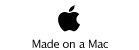JAVA, HTML5 & Chemistry Site

Other Writing

Last Update
02-Jan-2013

## Neural Network:

For example, there is the data like below. Almost all chemists are familiar to Mutiple Regression (MR).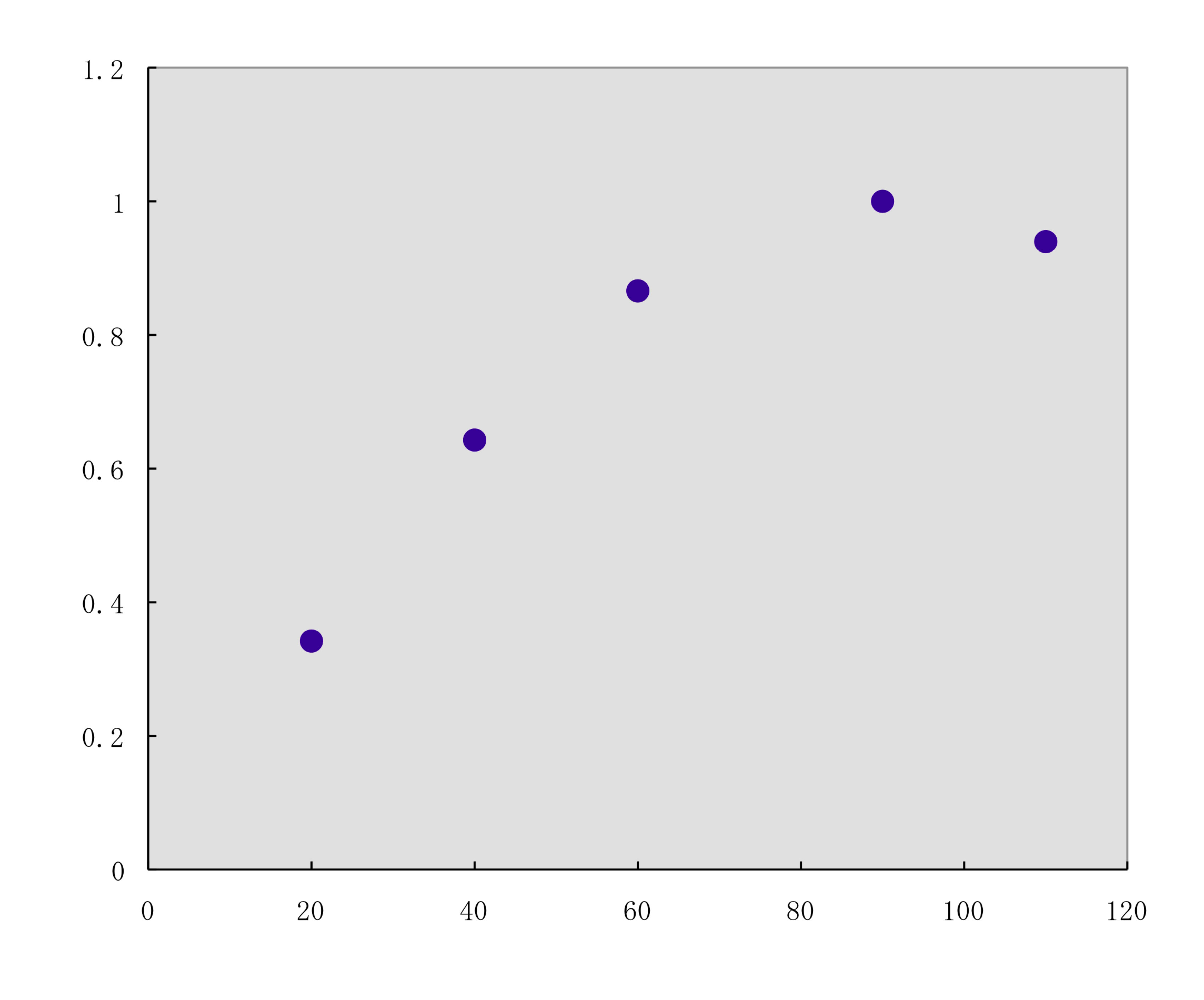If I explain MR very roughly, MR search Green line (Y=aX+b) so as the square pink length become minimum. The calculation is very easy and we can use MR in spread sheet. (Mac Excel does not support MR)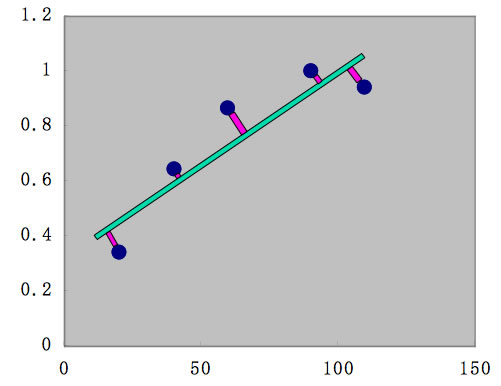Almost all Chemical area calculation, MR is enough. Actualy, above data point is part of Sin curve.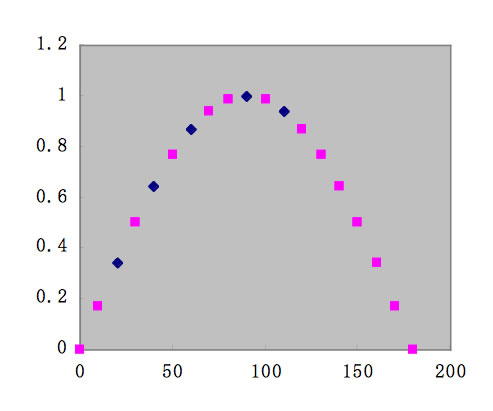If we want to treat this data, we need to apply non-linear MR method. For example, as the non-linear function,

f(y)=A1* / [1+exp(-(B1*X1+B2*1))＋
A2* / [1+exp(-(B3*X1+B4*1))＋
A3* / [1+exp(-(B5*X1+B6*1))

and determine A1-A3, B1-B6 coeeficients,
A1= -2.14 B1=-1.84 B2=0.30
A2= -1.01 B3=0.04   B4=0.31
A3=2.11   B5=-1.87  B6=1.57

Then prediction data point (blue small) become like below.f(y)=A1* / [1+exp(-(B1*X1+B2*1))＋
A2* / [1+exp(-(B3*X1+B4*1))＋
A3* / [1+exp(-(B5*X1+B6*1))

If we draw schematic diagram of this f(y) function, it become like below.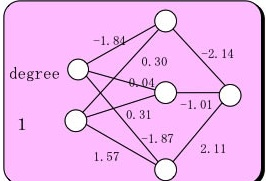it is very similar to neuron and synapse structure.The variable A1-A3, B1-B6 are thought as connective strength between neuron.

The function of f(y)'=A1* / [1+exp(-(B1*X1+B2*1)) is called Sigmoid function. This function change the curve at Threshold value θ, as the value of α.

This curve is very similar to Stimulation - Response curve in biology.So, this non-linear MR method is called neural network mothod.

## Over-Learning:

The computer never give up finding good function, so once ordered, it will find very good green curve like below.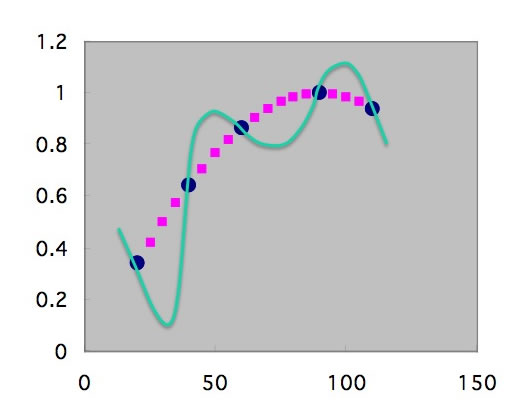The predited data that we want to is pink point, so learned point (Blue) is very good (R=1.00) but predicted point is Green line, so the error =(Green point - pink point) become very very large.

This over learning may happen when learning poinst (Blue) are small, and hidden layer neurons number are large.

To over come, what we can do is increase learning data poinst. If they increase, the curve can not go far from data point.The number of hidden layer neuron number means the number of sigmoid functions.

f(y)=A1* / [1+exp(-(B1*X1+B2*1))＋
A2* / [1+exp(-(B3*X1+B4*1))＋
A3* / [1+exp(-(B5*X1+B6*1))

If we increase sigmoid functions, we can trace complicated phenomena, but it means much more learning points.

## Interpolation, Extrapolation:

With case of Sin curve learning, when NN learn 0-90 degree, NN will answer blue dot. In this case, we use the term "NN have the good ability of Interpolation".Then what happen for over 90 degree point.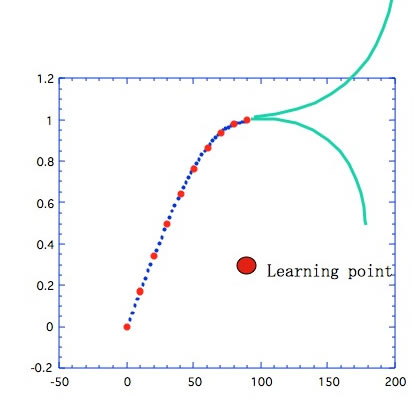NN can not predict Extrapolation data point. This nature of NN is so problematic. When I build NN for organic compounds' boiling point. Suppose, in learning DB, it exsit hydroxy groups containing compounds like OH*1 (ethanol) OH*2 (ethylene glycol) OH*3 (Glycerol). We can easily understand if compounds have OH*4, then it become Extrapolation. It is easy to find out. But if there is no data that have COOH*1, OH*1, even though COOH*1, COOH*2 .... data exist, COOH*1, OH*1 compound become Extrapolation. it become so hard to find out such combination Extrapolation.

So, the database become very important to bulid NN.